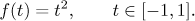# Solved Problems for Series: Series of Functions

Here we will show solved problems on various topics connected to series of functions. We start with convergence of sequences and series of functions, then we look at convergence of power series (radius of convergence etc.), do some Taylor series expansions and the opposite (summing up power series), and eventually we do some problems on Fourier series.
If you wish to simultaneously follow another text on series of functions in a separate window, click here for Theory and here for Methods Survey.

• Problem: Investigate convergence of the following sequence of functions: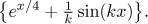• Problem: Investigate convergence of the following sequence of functions: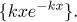• Problem: Investigate convergence of the following sequence of functions: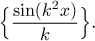• Problem: Investigate convergence of the following sequence of functions: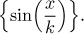• Problem: Investigate convergence of the following sequence of functions: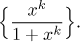• Problem: Investigate convergence of the following series of functions: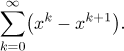• Problem: Investigate convergence of the following series of functions: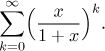• Problem: Investigate convergence of the following series of functions: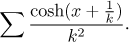• Problem: Investigate convergence of the following power series: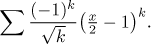• Problem: Investigate convergence of the following power series: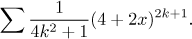• Problem: Investigate convergence of the following power series: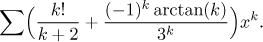• Problem: Expand the given function in a power (Taylor) series with the given center.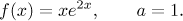• Problem: Expand the given function in a power (Taylor) series with the given center.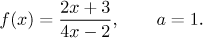• Problem: Expand the given function in a power (Taylor) series with the given center.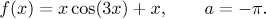• Problem: Expand the given function in a power (Taylor) series with the given center.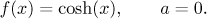• Problem: Expand the given function in a power (Taylor) series with the given center.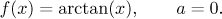• Problem: Expand the given function in a power (Taylor) series with the given center.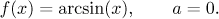• Problem: Sum up the following power series: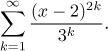• Problem: Sum up the following power series: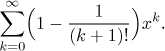• Problem: Sum up the following power series: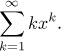• Problem: For the following function find its Fourier series, its sine Fourier series and its cosine Fourier series; for each series, determine its sum.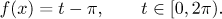• Problem: Find the Fourier series and its sum for the following function: